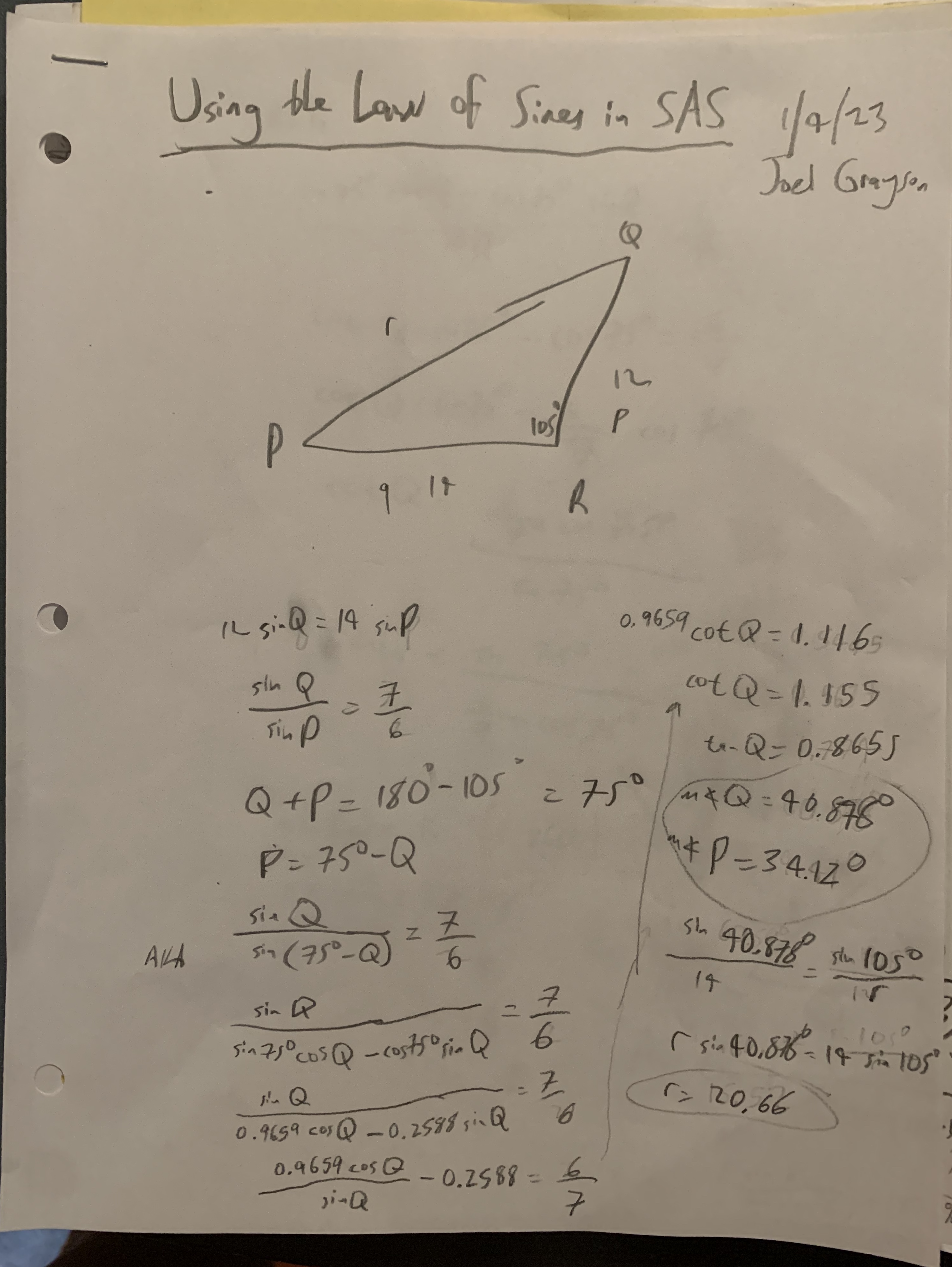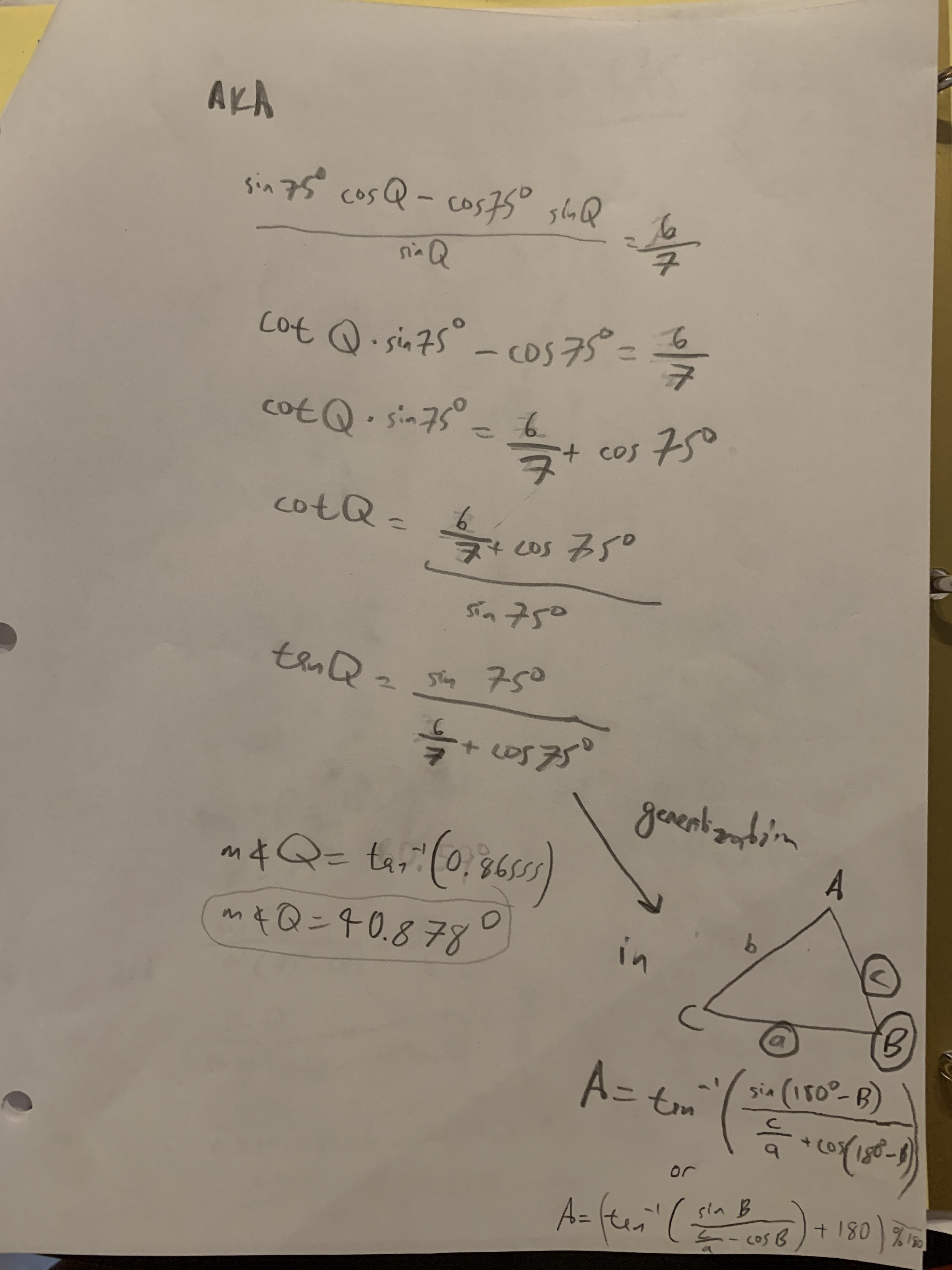# Joel's SAS Formula

Goal: find all the angles and sides of an SAS triangle using only the Law of Sines.

### Explanation

to play around with it in the Geometer's Sketchpad.

### Formula

This differs from the formula at the top because 180° needs to be added when the value is negative because tan
LaTeX: A=(tan^{-1}(\frac{sinB}{\frac{c}{a}-cosB})+180°) \bmod 180° in any triangle ABC.

### Derivation